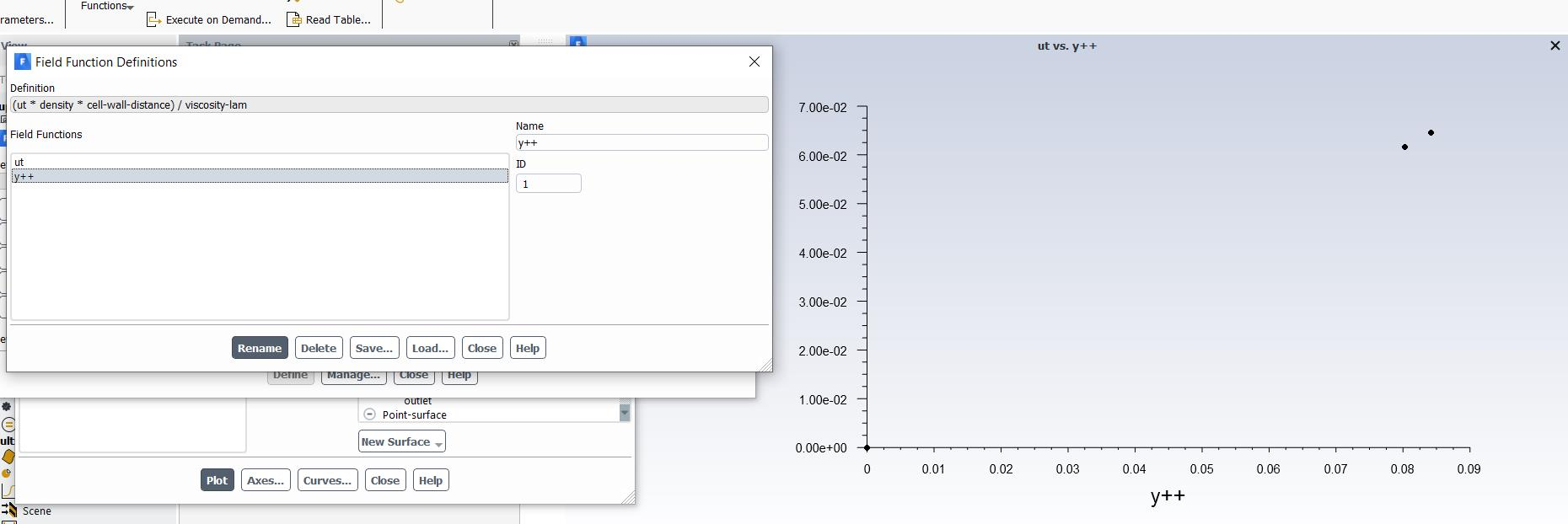# Define y+ in fluent

Hi every one

I want to plot Y+ vs U+ but the value of y+ is very small because I used y+=0.1 to 1 For LES model so how can I get a y+ values like 100 or 300

I read some of the discussions and some of them talk about define y+ how can I define it???

• Please post the questions in appropriate category. Moving this question to other category to get better response.

Moving to fluid dynamics.

You will need to coarsen the mesh to increase y+ value.

At the same time, for LES it is required to get Y+ as 1.

Regards,

Keyur

If this helps, please mark this post as 'Is Solution' to help others.

Guidelines on the Student Community

How to access ANSYS help links

ok, I will move it. but I need to plot some quantities with y+ so I need to get y+ at least =20

• edited May 18

You need to code the expression for Yplus so that you can plot along a line normal to the wall.

• You need to code the expression for Yplus so that you can plot along a line normal to the wall.

I know that but I don't have any idea about the code and how can i write it, because I'm a beginner user

the wall yplus read only first layer so If you have any information that can help me, I will be grateful

• Mr. rwoolhou I don't waste your time I try to put y+=1 but that not enough so I need the code to define y+ and I need more than one mesh, so I want to test the effect of y + on simulation

• edited May 18
You define a custom field function with the expression of yplus from manual which you later can plot along any line.
• I try to do that yplus=(y*ut /kinematic viscosity)  but her what y mean wall distance or coordinate y or what

• edited May 18
Wall distance
• thank you very much

•plot y+ vs ut

that what I got it

• edited May 19

Looks good or not? Just remember that Cell Wall Distance is the distance to the next wall.

• the value of y+ is also small so not good I need a big value like 20 or 30

• edited May 20

Then use a coarser mesh.

• Mr.rwoolhou I know courser mesh gives a large value of y+ but I ask if I can get a large value of y+ from  a fine mesh